Trigonometry : Sum, Difference, and Product Identities

Example Questions

← Previous 1 3

Example Question #1 : Sum, Difference, And Product Identities

True or false: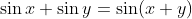.

False

True

Cannot be determined

False

Explanation:

The sum of sines is given by the formula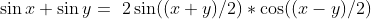.

Example Question #2 : Sum, Difference, And Product Identities

True or false: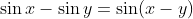.

False

True

Cannot be determined

False

Explanation:

The difference of sines is given by the formula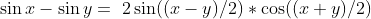.

Example Question #1 : Complete A Proof Using Sums, Differences, Or Products Of Sines And Cosines

True or false: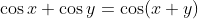.

Cannot be determined

False

True

False

Explanation:

The sum of cosines is given by the formula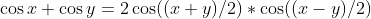.

Example Question #2 : Complete A Proof Using Sums, Differences, Or Products Of Sines And Cosines

True or false: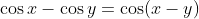.

Cannot be determined

True

False

False

Explanation:

The difference of cosines is given by the formula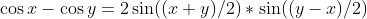.

Example Question #5 : Sum, Difference, And Product Identities

Which of the following correctly demonstrates the compound angle formula?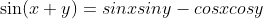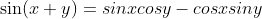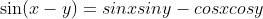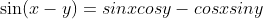Explanation:

The compound angle formula for sines states that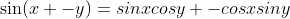.

Example Question #6 : Sum, Difference, And Product Identities

Which of the following correctly demonstrates the compound angle formula?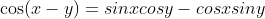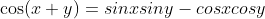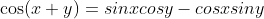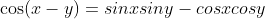Explanation:

The compound angle formula for cosines states that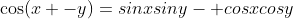.

Example Question #7 : Sum, Difference, And Product Identities

Simplify by applying the compound angle formula: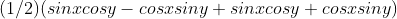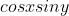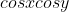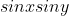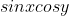Explanation:

Using the compound angle formula, we can rewrite each half of the non-coefficient terms in the given expression. Given thatand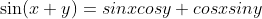, substitution yields the following: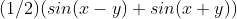This is the formula for the product of sine and cosine,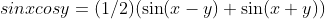.

Example Question #8 : Sum, Difference, And Product Identities

Simplify by applying the compound angle formula: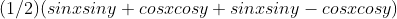Explanation:

Using the compound angle formula, we can rewrite each half of the non-coefficient terms in the given expression. Given that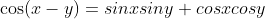and, substitution yields the following: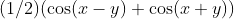This is the formula for the product of two cosines,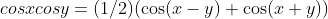.

Example Question #9 : Sum, Difference, And Product Identities

Using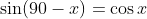and the formula for the sum of two sines, rewrite the sum of cosine and sine: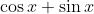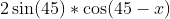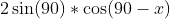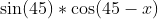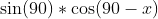Explanation:

Substitute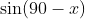for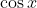: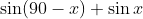Apply the formula for the sum of two sines,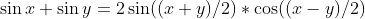: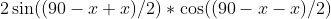Example Question #10 : Sum, Difference, And Product Identities

Usingand the formula for the difference of two sines, rewrite the difference of cosine and sine: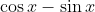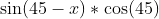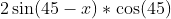Explanation:

Substitutefor: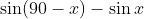Apply the formula for the difference of two sines,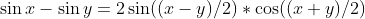.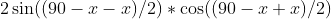← Previous 1 3